Publicité

# QB104541.pdf

11 Aug 2022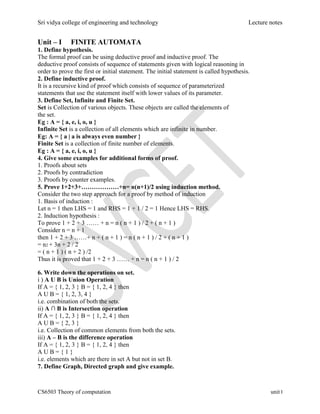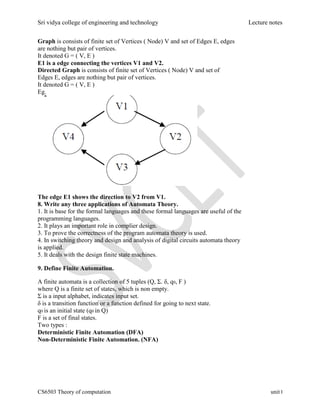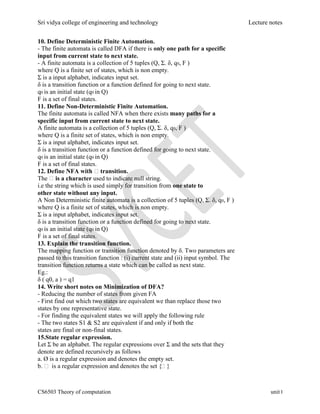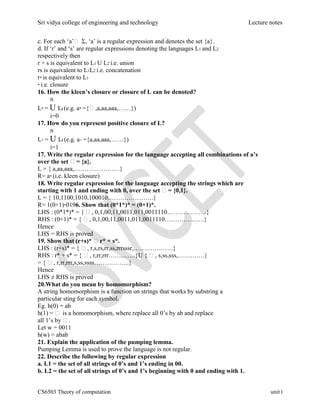Publicité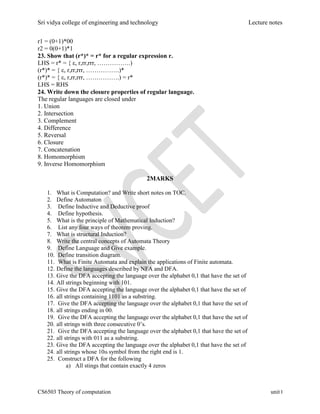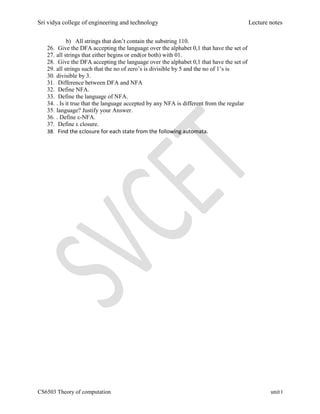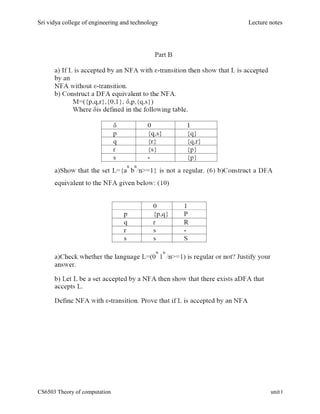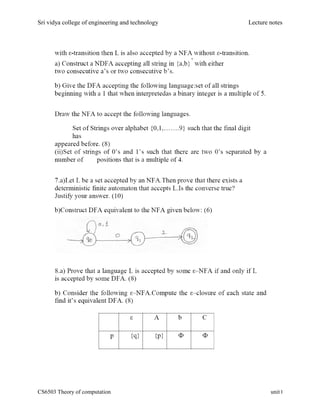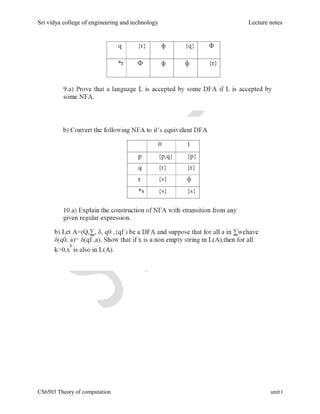Prochain SlideShare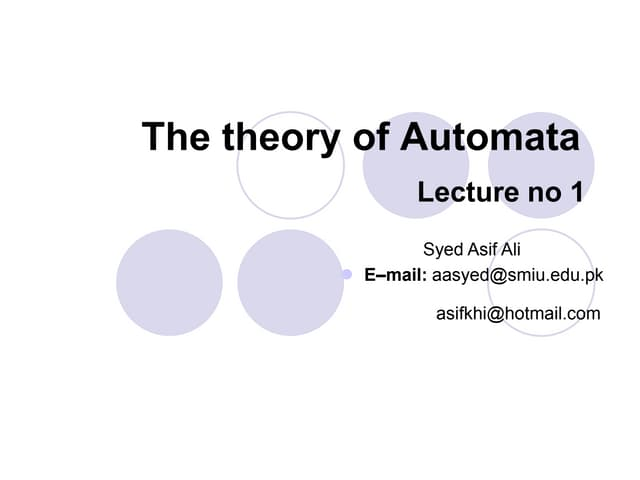Automata
Chargement dans ... 3
1 sur 9
Publicité

### QB104541.pdf

1. Sri vidya college of engineering and technology Lecture notes CS6503 Theory of computation unit I Unit – I FINITE AUTOMATA 1. Define hypothesis. The formal proof can be using deductive proof and inductive proof. The deductive proof consists of sequence of statements given with logical reasoning in order to prove the first or initial statement. The initial statement is called hypothesis. 2. Define inductive proof. It is a recursive kind of proof which consists of sequence of parameterized statements that use the statement itself with lower values of its parameter. 3. Define Set, Infinite and Finite Set. Set is Collection of various objects. These objects are called the elements of the set. Eg : A = { a, e, i, o, u } Infinite Set is a collection of all elements which are infinite in number. Eg: A = { a | a is always even number } Finite Set is a collection of finite number of elements. Eg : A = { a, e, i, o, u } 4. Give some examples for additional forms of proof. 1. Proofs about sets 2. Proofs by contradiction 3. Proofs by counter examples. 5. Prove 1+2+3+………………+n= n(n+1)/2 using induction method. Consider the two step approach for a proof by method of induction 1. Basis of induction : Let n = 1 then LHS = 1 and RHS = 1 + 1 / 2 = 1 Hence LHS = RHS. 2. Induction hypothesis : To prove 1 + 2 + 3 …… + n = n ( n + 1 ) / 2 + ( n + 1 ) Consider n = n + 1 then 1 + 2 + 3 ……+ n + ( n + 1 ) = n ( n + 1 ) / 2 + ( n + 1 ) = n2 + 3n + 2 / 2 = ( n + 1 ) ( n + 2 ) /2 Thus it is proved that 1 + 2 + 3 …… + n = n ( n + 1 ) / 2 6. Write down the operations on set. i ) A U B is Union Operation If A = { 1, 2, 3 } B = { 1, 2, 4 } then A U B = { 1, 2, 3, 4 } i.e. combination of both the sets. ii) A ∩ B is Intersection operation If A = { 1, 2, 3 } B = { 1, 2, 4 } then A U B = { 2, 3 } i.e. Collection of common elements from both the sets. iii) A – B is the difference operation If A = { 1, 2, 3 } B = { 1, 2, 4 } then A U B = { 1 } i.e. elements which are there in set A but not in set B. 7. Define Graph, Directed graph and give example.
2. Sri vidya college of engineering and technology Lecture notes CS6503 Theory of computation unit I Graph is consists of finite set of Vertices ( Node) V and set of Edges E, edges are nothing but pair of vertices. It denoted G = ( V, E ) E1 is a edge connecting the vertices V1 and V2. Directed Graph is consists of finite set of Vertices ( Node) V and set of Edges E, edges are nothing but pair of vertices. It denoted G = ( V, E ) Eg. The edge E1 shows the direction to V2 from V1. 8. Write any three applications of Automata Theory. 1. It is base for the formal languages and these formal languages are useful of the programming languages. 2. It plays an important role in complier design. 3. To prove the correctness of the program automata theory is used. 4. In switching theory and design and analysis of digital circuits automata theory is applied. 5. It deals with the design finite state machines. 9. Define Finite Automation. A finite automata is a collection of 5 tuples (Q, Σ. δ, q0, F ) where Q is a finite set of states, which is non empty. Σ is a input alphabet, indicates input set. δ is a transition function or a function defined for going to next state. q0 is an initial state (q0 in Q) F is a set of final states. Two types : Deterministic Finite Automation (DFA) Non-Deterministic Finite Automation. (NFA)
3. Sri vidya college of engineering and technology Lecture notes CS6503 Theory of computation unit I 10. Define Deterministic Finite Automation. - The finite automata is called DFA if there is only one path for a specific input from current state to next state. - A finite automata is a collection of 5 tuples (Q, Σ. δ, q0, F ) where Q is a finite set of states, which is non empty. Σ is a input alphabet, indicates input set. δ is a transition function or a function defined for going to next state. q0 is an initial state (q0 in Q) F is a set of final states. 11. Define Non-Deterministic Finite Automation. The finite automata is called NFA when there exists many paths for a specific input from current state to next state. A finite automata is a collection of 5 tuples (Q, Σ. δ, q0, F ) where Q is a finite set of states, which is non empty. Σ is a input alphabet, indicates input set. δ is a transition function or a function defined for going to next state. q0 is an initial state (q0 in Q) F is a set of final states. 12. Define NFA with transition. The is a character used to indicate null string. i.e the string which is used simply for transition from one state to other state without any input. A Non Deterministic finite automata is a collection of 5 tuples (Q, Σ. δ, q0, F ) where Q is a finite set of states, which is non empty. Σ is a input alphabet, indicates input set. δ is a transition function or a function defined for going to next state. q0 is an initial state (q0 in Q) F is a set of final states. 13. Explain the transition function. The mapping function or transition function denoted by δ. Two parameters are passed to this transition function : (i) current state and (ii) input symbol. The transition function returns a state which can be called as next state. Eg.: δ ( q0, a ) = q1 14. Write short notes on Minimization of DFA? - Reducing the number of states from given FA - First find out which two states are equivalent we than replace those two states by one representative state. - For finding the equivalent states we will apply the following rule - The two states S1 & S2 are equivalent if and only if both the states are final or non-final states. 15.State regular expression. Let Σ be an alphabet. The regular expressions over Σ and the sets that they denote are defined recursively as follows a. Ø is a regular expression and denotes the empty set. b.  is a regular expression and denotes the set { }
4. Sri vidya college of engineering and technology Lecture notes CS6503 Theory of computation unit I c. For each ‘a’ Σ, ‘a’ is a regular expression and denotes the set {a}. d. If ‘r’ and ‘s’ are regular expressions denoting the languages L1 and L2 respectively then r + s is equivalent to L1 U L2 i.e. union rs is equivalent to L1L2 i.e. concatenation r* is equivalent to L1 * i.e. closure 16. How the kleen’s closure or closure of L can be denoted? n L* = U Li (e.g. a* ={,a,aa,aaa,……}) i=0 17. How do you represent positive closure of L? n L+ = U Li (e.g. a+ ={a,aa,aaa,……}) i=1 17. Write the regular expression for the language accepting all combinations of a’s over the set = {a}. L = { a,aa,aaa,………………….} R= a* (i.e. kleen closure) 18. Write regular expression for the language accepting the strings which are starting with 1 and ending with 0, over the set = {0,1}. L = { 10,1100,1010,100010………………….} R= 1(0+1)*0196. Show that (0*1*)* = (0+1)*. LHS : (0*1*)* = { , 0,1,00,11,0011,011,0011110……………….} RHS : (0+1)* = { , 0,1,00,11,0011,011,0011110……………….} Hence LHS = RHS is proved 19. Show that (r+s)* r* + s*. LHS : (r+s)* = { , r,s,rs,rr,ss,rrrsssr,……………….} RHS : r* + s* = { , r,rr,rrr………….}U { , s,ss,sss,………….} = { , r,rr,rrr,s,ss,ssss……………..} Hence LHS ≠ RHS is proved 20.What do you mean by homomorphism? A string homomorphism is a function on strings that works by substring a particular sting for each symbol. Eg. h(0) = ab h(1) =  is a homomorphism, where replace all 0’s by ab and replace all 1’s by . Let w = 0011 h(w) = abab 21. Explain the application of the pumping lemma. Pumping Lemma is used to prove the language is not regular. 22. Describe the following by regular expression a. L1 = the set of all strings of 0’s and 1’s ending in 00. b. L2 = the set of all strings of 0’s and 1’s beginning with 0 and ending with 1.
5. Sri vidya college of engineering and technology Lecture notes CS6503 Theory of computation unit I r1 = (0+1)*00 r2 = 0(0+1)*1 23. Show that (r*)* = r* for a regular expression r. LHS = r* = { ε, r,rr,rrr, …………….) (r*)* = { ε, r,rr,rrr, …………….)* (r*)* = { ε, r,rr,rrr, …………….) = r* LHS = RHS 24. Write down the closure properties of regular language. The regular languages are closed under 1. Union 2. Intersection 3. Complement 4. Difference 5. Reversal 6. Closure 7. Concatenation 8. Homomorphism 9. Inverse Homomorphism 2MARKS 1. What is Computation? and Write short notes on TOC. 2. Define Automaton 3. Define Inductive and Deductive proof 4. Define hypothesis. 5. What is the principle of Mathematical Induction? 6. List any four ways of theorem proving. 7. What is structural Induction? 8. Write the central concepts of Automata Theory 9. Define Language and Give example. 10. Define transition diagram. 11. What is Finite Automata and explain the applications of Finite automata. 12. Define the languages described by NFA and DFA. 13. Give the DFA accepting the language over the alphabet 0,1 that have the set of 14. All strings beginning with 101. 15. Give the DFA accepting the language over the alphabet 0,1 that have the set of 16. all strings containing 1101 as a substring. 17. Give the DFA accepting the language over the alphabet 0,1 that have the set of 18. all strings ending in 00. 19. Give the DFA accepting the language over the alphabet 0,1 that have the set of 20. all strings with three consecutive 0’s. 21. Give the DFA accepting the language over the alphabet 0,1 that have the set of 22. all strings with 011 as a substring. 23. Give the DFA accepting the language over the alphabet 0,1 that have the set of 24. all strings whose 10th symbol from the right end is 1. 25. Construct a DFA for the following a) All stings that contain exactly 4 zeros
6. Sri vidya college of engineering and technology Lecture notes CS6503 Theory of computation unit I b) All strings that don’t contain the substring 110. 26. Give the DFA accepting the language over the alphabet 0,1 that have the set of 27. all strings that either begins or end(or both) with 01. 28. Give the DFA accepting the language over the alphabet 0,1 that have the set of 29. all strings such that the no of zero’s is divisible by 5 and the no of 1’s is 30. divisible by 3. 31. Difference between DFA and NFA 32. Define NFA. 33. Define the language of NFA. 34. . Is it true that the language accepted by any NFA is different from the regular 35. language? Justify your Answer. 36. . Define ε-NFA. 37. Define ε closure. 38. Find the εclosure for each state from the following automata.
7. Sri vidya college of engineering and technology Lecture notes CS6503 Theory of computation unit I
8. Sri vidya college of engineering and technology Lecture notes CS6503 Theory of computation unit I
9. Sri vidya college of engineering and technology Lecture notes CS6503 Theory of computation unit I
Publicité